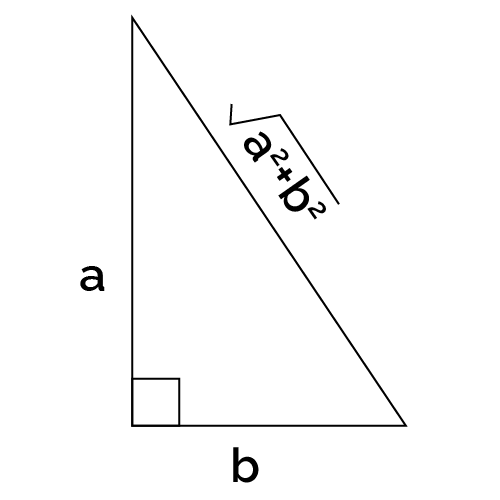# Perimeter of a Right Triangle Calculator

Created by Komal Rafay
Reviewed by Davide Borchia
Last updated: Feb 02, 2023

The perimeter of a right triangle calculator is a quick and witty tool that allows you to determine the perimeter of a right-angle triangle based on the length of its sides based on various options.
We have many exciting things in store for all you geometry enthusiasts out there:

• What is the perimeter of a right triangle;
• The formula for right triangle perimeter; and
• How to calculate the perimeter of a right-angled triangle.

## What is the perimeter of a right triangle

As you may already know, the perimeter of an object or shape is the sum of all its sides. In this case, the perimeter of a triangle is the sum of its three sides.

So how is the perimeter of a right triangle different? In reality, it is not. It is still the sum of all three sides. The only difference is that the triangle is specific to right triangles only.

## Perimeter of a right triangle calculator

The perimeter of a right triangle calculator is your one-stop tool that efficiently determines the perimeter of the infamous right-angled triangle.
But the best part is that you can do that two ways. One is when you don't have the hypotenuse, and the other is when you have the hypotenuse.
The tool gives you a choice at the top.
Choose the formula you want to use,

1. Given the catheti; or
2. Given all three sides.

For the first option, when only the perpendicular and the base, also known as catheti, are given, the formula uses the Pythagoras theorem to identify the hypotenuse and then calculate the perimeter.

• Input the perpendicular;
• Input the base of your right triangle; and
• The result is the perimeter of the right triangle.

All three sides of the right triangle are known for the second option. So, here:

• Input the perpendicular;
• Input the base;
• Input the hypotenuse; and
• The result is the perimeter of your right triangle.

## Formula for right triangle perimeter

There are two formulas that can be used to determine the perimeter of a triangle depending on the sides.

### 1. Using only the two sides (catheti):

$P = a + b + \sqrt {(a^2+b^2)}$

So using this formula comes in handy when you do not have the hypotenuse value, as the Pythagoras equation can determine it.

### 2. Using all three sides:

$P = a + b + h$

where:

• $P$ - The perimeter;
• $a$ - The perpendicular;
• $b$ - The base; and
• $h$ - The hypotenuse.

This formula is relatively simple, requiring summing up all three sides. We can do that at our fingertips.

These formulas are here to help you understand how to find the perimeter of a right triangle.

## Omni's perimeter of a triangle calculators

Other tools at Omni calculate the perimeters of triangles based on different types and information available.

## FAQ

### What is the perimeter of a right triangle?

The perimeter of a right triangle is no different than the perimeter of any other shape. It is the sum of all its sides, like a circumference. The perimeter of a right triangle is specifically for right-angled triangles only. It is the sum of the three sides, the base, perpendicular, and the hypotenuse.

### How can I calculate the perimeter of a right angled triangle?

The perimeter of a right-angled triangle can be calculated in two ways:

When you have only the two sides of the right triangle, the base, and perpendicular, then use the formula:
P = a + b + √(a² + b²)

When you have all the three sides of the right triangle, use the formula:
P = a + b + h

where:

• P - The perimeter;
• a - The perpendicular;
• b - The base; and
• h - The hypotenuse.

### What is the perimeter, if catheti are 8 and 9 cm?

The perimeter is 29.04 cm if the catheti, i.e., the perpendicular and the base, are 8 and 9 cm.

The formula to determine the perimeter of a right triangle, when only two sides are known, is:

P = a + b + √(a² + b²)

where:

• P - The perimeter;
• a - The perpendicular; and
• b - The base.
Komal Rafay
Given
CathetiBase
cm
Perpendicular
cm
Perimeter
cm
People also viewed…

### Car vs. Bike

Everyone knows biking is fantastic, but only this Car vs. Bike Calculator turns biking hours into trees! 🌳

### Coordinate grid

Our coordinate grid calculator can generate a grid of coordinates in a 2D space in all the possible regular grids.

### Fundamental counting principle

The fundamental counting principle calculator will help you find out how many options you have for choosing up to ten characteristics of whatever it is you're dealing with.

### Pizza size

This calculator will help you make the most delicious choice when ordering pizza.